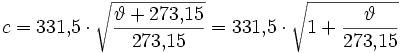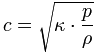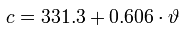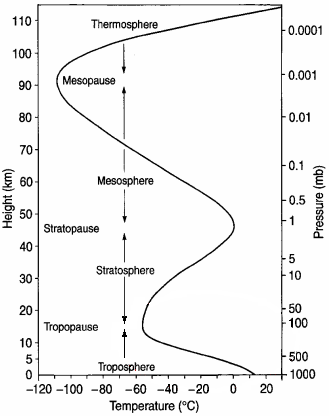# Is sound directly proportional to temperatureTable: The clear effect of temperature
The air density, the speed of sound and the characteristic
Acoustic impedance as a function of the temperature of the air

 temperature the air ϑ in ° C Speed ​​of soundc in m / s Time per 1 mΔt in ms / m Airtightnessρ in kg / m3 Acoustic impedance of air Z0 in Ns / m3 +40 354,94 2,817 1,1272 400,0 +35 352,17 2,840 1,1455 403,4 +30 349,29 2,864 1,1644 406,7 +25 346,39 2,888 1,1839 410,0 +20 343,42 2,912 1,2041 413,5 +15 340,51 2,937 1,2250 417,1 +10 337,54 2,963 1,2466 420,8 +5 334,53 2,990 1,2690 424,5 ±0 331,50 3,017 1,2920 428,3 −5 328,44 3,044 1,3163 432,3 −10 325,35 3,073 1,3413 436,4 −15 322,23 3,103 1,3673 440,6 −20 319,09 3,134 1,3943 444,9 −25 315,91 3,165 1,4224 449,4

ϑ = Temperature in ° C, c = Speed ​​of sound, ρ = Density,
Z0 = ρ ·c = Characteristic resistance (specific acoustic impedance) of air
Sound pressure p = √ (I. × Z0) and sound intensity I. = p² /Z0      ρ = 101325 / (287,058 · (273,15 + ϑ))
Standard air pressure p0 = 101325 Pa, specific gas constant R. = 287.058 J / kg · K.

Only because the air temperature decreases with altitude does the speed of sound also decrease.

 As a reference value, the acoustic characteristic impedance of the surrounding air (acoustic characteristic impedance) is uniform with the round value Z0 = 400 N·s / m³ (Pa·s / m) in physics (acoustics) - without going into a specific temperature. With this round value, the "sound level" as a decibel value, i.e. the sound pressure level, exactly matches the sound intensity level.The reference acoustic impedance Z0 = ρ · c = 400 N · s / m³ is calculated from the values ​​of the hearing threshold of sound pressure p0 = 20 µPa (µN / m²) and the sound intensity I.0 = 1 pW / m². The sound pressure level and the sound intensity level are therefore with a characteristic sound impedance ofZ0 = 400 N·s / m³ absolutely identical.

 The speed of sound in air cis determined by the medium air and is not of the amplitude, the frequency and the wavelength of Sound dependent.With an ideal gas, the speed of sound depends only on the temperature and independent of the gas pressure. This dependence therefore also applies to air that is in good Approximation can be viewed as an ideal gas.Note: The speed of sound changes significantly with temperature, something with the humidity - but Not with our air pressure. The statement "sound pressure at sea level" is incorrect and misleading. However, the temperature specification is absolutely necessary for this.

 The mean air pressure at sea level is 101,325 Pa. However, this information is insignificant for the speed of sound. For this we always need the temperature information.

Speed ​​of sound in air

 Simply enter the value on the left or right. The calculator works in both directions of the ↔ Character.When entering a decimal, the is Point to use.

 The speed of sound in German textbooks is at 0 ° Celsius 331.5 m / s. At 20 ° Celsius the speed of sound is then 343.42 m / s, rounded 343 m / s. At 0 ° Celsius, the speed of sound in US textbooks is 331.3 m / s. At 20 ° Celsius the speed of sound is then 343.21 m / s, rounded 343 m / s.

The effect of temperature

 The air densityis: ρ = p / (R. ·T) in kg / m3, Air pressure = p, Gas constant = R., Temperature in Kelvin = T  The individual gas constant R. for dry air is: R. = 287.058 years / kg · K  with energy Joule (J) = Newton · Meter = N m; T in Kelvin = temperature in ° C + 273.15.  Atmospheric pressure p0 = 101325 Pa = 1013.25 mbar = 1013.25 hPa and R. = 287.058 J / kg · K.  At T0 = 273.15 K (0 ° C) (normal conditions) the air density is: ρ0 = 101325 / (287,058 · 273.15) = 1.2922 kg / m3.  At T25 = 298.15 K (25 ° C) (standard conditions) the air density is: ρ25 = 101325 / (287,058 · 298.15) = 1.184 kg / m3.  It is also common T20 = 293.15 K ↔ 20 ° C and where the air density is ρ = 1.2041 kg / m3.  As you can see, these quantities are clearly temperature-dependent.  The speed of sound in air is temperature dependent:ϑ (theta) is the temperature in ° C. Z0 = ρ0 · c0 Here is Z0 the acoustic impedance of air and c is the speed of sound.  In SI units, when the air is dry and 20 ° C (68 ° F) is the speed of sound c = 343 m / s. This corresponds to 1235 km / h (not km / h), 767 mph, 1125 ft / s or 666 knots.

 Reason: The static air pressure p_ and the density ρ the air are at the same Temperature proportional to each other. The relationship p / ρ is always constant; on a high mountain or even at sea level. When calculating the speed of sound forget the air pressure, but be sure to pay attention to the important temperature. The speed of sound changes with altitude only because of the change in air temperature!Adiabatic exponent (isentropic exponent) κ (kappa) = cp/ cVGenerally applies with sufficient
Accuracy as Formula for the speed of sound (Speed ​​of propagation) of
Air in m / s depending on the temperature ϑ (theta) in ° C:in m / s.

This results in, for example ϑ = 20 ° C a speed of sound of c = 331,3 + (0,606 · 20)
= 343.42 m / s. Often the formula is sufficient: c ≈ 331 + (0.6 · 20) = 343 m / s.

 A temperature change of 1 ° C means 60 cm / s Change in the speed of sound.

Formula: temperature of the air ϑ = 273.15 (0.00000909981 c2 − 1) in ° C.
 There is a useful formula (rule of thumb) to calculate the temperature ϑ (° C),when the speed of sound c (m / s) in air is known. Formula: temperature of the airϑ ≈ (c − 331) / 0,6 in ° C. The speed of sound can be calculated more precisely using the following formula. Speed ​​of soundin m / s; Temperature ϑ in ° C.Air temperature ϑ ° C = 273.15 · (0,000009099822780951 · c² - 1) in ° C. Speed ​​of sound c = 331.5 · sqrt (1+ (ϑ ° C / 273.15))

Conversion: speed of sound and air temperature

 Simply enter the value on the left or right. The calculator works in both directions of the ↔ Character.

 The speed of sound c depends on the temperature of the air and not on the air pressure p! The air humidity has a small negligible effect on the speed of sound.Note: The air pressure and the air density are proportional to each other at the same temperature. Always is the relationship p_ / ρ = constant. Rho is the density ρ and p_ is the static one Air pressure. The air pressure is therefore not included in the calculation of the speed of sound in air.

 Acousticians and sound protectors ("noise fighters") need the sound intensity and the sound power - however, sound engineers and sound designers ("ear people") hardly need this amount of sound energy. Therefore, one should rather take care of the sound field size, the alternating sound pressure and the level (sound pressure level SPL) as an effect on the eardrums of the hearing and on the diaphragms of the microphones, as well as the corresponding audio voltage or its voltage level.

Sound pressure and sound power - effect and cause

 The decisive factor for the sound sensation in the ear is that from the Rapidly changing alternating pressure resulting from air particle movement of sound waves, which is called sound pressure.

Converter: Celsius to Fahrenheit and Fahrenheit to Celsius

 Simply enter the value on the left or right. The calculator works in both directions of the ↔ Character.
Formulas for converting from and to degrees Celsius

 From Celsius to x degrees From x degrees to Celsius Fahrenheit ° F = ° C × 9/5 + 32 ° C = (° F - 32) × 5/9 Kelvin K = ° C + 273.15 ° C = K - 273.15 Rankine ° R = (° C + 273.15) × 9/5 ° C = (° R - 491.67) × 5/9 Delisle ° De = (100 - ° C) × 3/2 ° C = 100 - ° De × 2/3 Newton ° N = ° C × 33/100 ° C = ° N × 100/33 Réaumur ° Ré = ° C × 4/5 ° C = ° Ré × 5/4 Romer ° Rø = ° C × 21/40 + 7,5 ° C = (° Rø - 7.5) × 40/21

Formulas for converting from and to degrees Fahrenheit

 From Fahrenheit to x degrees From x degrees to Fahrenheit Celsius ° C = (° F - 32) × 5/9 ° F = ° C × 9/5 + 32 Kelvin K = (° F + 459.67) × 5/9 ° F = K × 9/5 − 459,67 Rankine ° R = ° F + 459.67 ° F = ° R - 459.67 Delisle ° De = (212 - ° F) × 5/6 ° F = 212 - ° De × 6/5 Newton ° N = (° F - 32) × 11/60 ° F = ° N × 60/11 + 32 Réaumur ° Ré = (° F - 32) × 4/9 ° F = ° Ré × 9/4 + 32 Romer ° Rø = (° F - 32) × 7/24 + 7.5 ° F = (° Rø - 7.5) × 24/7 + 32

 Vertical profile of the temperature of the atmosphere in June at 45 ° northTemperature as a function of altitude (air pressure)The question of the exact speed of sound cannot be answered. To do this, you always need to specify the temperature and humidity, but not the air pressure. The specification of the speed of sound above "sea level" or sea level is nonsense, because only the temperature is significant and not the height above sea level.

Density of water ρ (rho) (pure and air-free)
at normal pressure
p0 = 101325 Pa.
Temperature between 0 ° C and 100 ° C

 Temperature in ° C - ρ in kg / m³ Temperature in ° C - ρ in kg / m³ 0 918.00 (ice cream)  0        999,84  1        999,90  2        999,94  3        999,96     4        999,97 ●  5        999,96  6        999,94  7        999,90  8        999,85  9        999,78 10        999,70 11        999,60 12        999,50 13        999,38 14        999,24 15        999,10 16        998,94 17        998,77 18        998,59 19        998,40 20        998,20 21        997,99 22        997,77 23        997,54 24        997,29 25        997,04 26        996,78 27        996,51 28        996,23 29        995,94 30        995,64 31        995,34 32        995,02 33        994,70 34        994,37 35        994,03 36        993,68 37        993,32 38        992,96 39        992,59 40        992,21 45        990,21 50        988,03 55        985,69 60        983,19 65        980,55 70        977,76 75        974,84 80        971,79 85        968,61 90        965,30 95        961,88100        958,35   Water vapor, 101325 Pa:100         0,5

 The dependence of the water density on the pressure is relatively low. For every 1 bar = 100,000 Pa pressure increase, the density increases by around 0.046 kg / m³. Normal air pressure fluctuations therefore have practically no influence on the density of the water.